Elliott Sound Products AN-001

# Precision Rectifiers

Rod Elliott (ESP)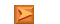App. Notes IndexMain Index

First and Second Rules of Opamps

To be able to understand much of the following, the basic rules of opamps need to be firmly embedded in the skull of the reader.  I came up with these many years ago, and - ignoring small errors caused by finite gain, input and output impedances - all opamp circuits make sense once these rules are understood.  They are also discussed in the article Designing With Opamps in somewhat greater detail.  Highly recommended if you are in the least bit unsure.

The two rules are as follows ...
1. An opamp will attempt to make both inputs exactly the same voltage (via the feedback path)
2. If it cannot achieve #1, the output will assume the polarity of the most positive input

These two rules describe everything an opamp does in any circuit, with no exceptions ... provided that the opamp is operating within its normal parameters.  This means power supply voltage(s) must be within specifications, signal voltage is within the allowable range, and load impedance is equal to or greater than the minimum specified.  The signal frequency must also be low enough to ensure that the opamp can perform normally for the chosen gain.  For most cheap opamps, a gain of 100 with a frequency of 1kHz should be considered the maximum allowable, since the opamp's open loop gain may not be high enough to accommodate higher gain or frequency.

Armed with these rules and a basic understanding of Ohm's Law and analogue circuitry, it is possible to figure out what any opamp circuit will do under all normal operating conditions.  Needless to say, the rules no longer apply if the opamp itself is faulty, or is operating outside its normal parameters (as discussed briefly above).

Half Wave Precision Rectifier

There are many applications for precision rectifiers, and while most are suitable for use in audio circuits, I thought it best to make this the first ESP Application Note.  While some of the existing projects in the audio section have a rather tenuous link to audio, this information is more likely to be used for instrumentation purposes than pure audio applications.

Typically, the precision rectifier is not commonly used to drive analogue meter movements, as there are usually much simpler methods to drive floating loads such as meters.  Precision rectifiers are more common where there is some degree of post processing needed, feeding the side chain of compressors and limiters, or to drive digital meters.

There are several different types of precision rectifier, but before we look any further, it is necessary to explain what a precision rectifier actually is.  In its simplest form, a half wave precision rectifier is implemented using an opamp, and includes the diode in the feedback loop.  This effectively cancels the forward voltage drop of the diode, so very low level signals (well below the diode's forward voltage) can still be rectified with minimal error.

The most basic form is shown in Figure 1, and while it does work, it has some serious limitations.  The main one is speed - it will not work well with high frequency signals.  To understand the reason, we need to examine the circuit closely.  This knowledge applies to all subsequent circuits, and explains the reason for the apparent complexity.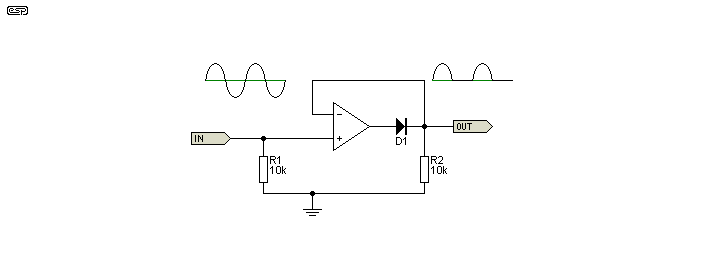Figure 1 - Basic Precision Half Wave Rectifier

For a low frequency positive input signal, 100% negative feedback is applied when the diode conducts.  The forward voltage is effectively removed by the feedback, and the inverting input follows the positive half of the input signal almost perfectly.  When the input signal becomes negative, the opamp has no feedback at all, so the output pin of the opamp swings negative as far as it can.  Assuming 15V supplies, that means perhaps -14V on the opamp output.

When the input signal becomes positive again, the opamp's output voltage will take a finite time to swing back to zero, then to forward bias the diode and produce an output.  This time is determined by the opamp's slew rate, and even a very fast opamp will be limited to low frequencies - especially for low input levels.  The test voltage for the waveforms shown was 20mV at 1kHz.  Although the circuit does work very well, it is limited to relatively low frequencies (less than 10kHz) and only becomes acceptably linear above 10mV or so (opamp dependent).

Note the oscillation at the rectified output.  This is (more or less) real, and was confirmed with an actual (as opposed to simulated) circuit.  This is the result of the opamp becoming open-loop with negative inputs.  In most cases it is not actually a problem.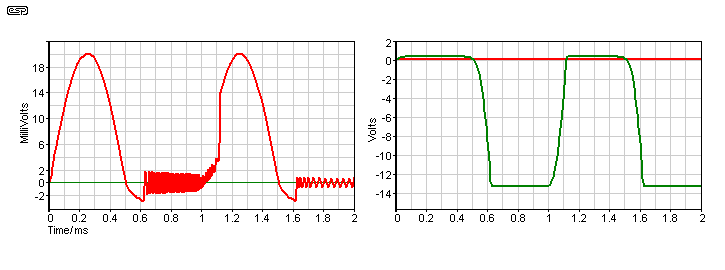Figure 2 - Rectified Output and Opamp Output

Figure 2 shows the output waveform (left) and the waveform at the opamp output (right).  The recovery time is obvious on the rectified signal, but the real source of the problem is quite apparent from the huge voltage swing before the diode.  While this is of little consequence for high level signals, it causes considerable non-linearity for low levels, such as the 20mV signal used in these examples.

The circuit is improved by reconfiguration, as shown in Figure 3.  The additional diode prevents the opamp's output from swinging to the negative supply rail, and low level linearity is improved dramatically.  A 2mV (peak) signal is rectified with reasonably good accuracy.  Although the opamp still operates open-loop at the point where the input swings from positive to negative or vice versa, the range is limited by the diode and resistor.  Recovery time is therefore a great deal shorter.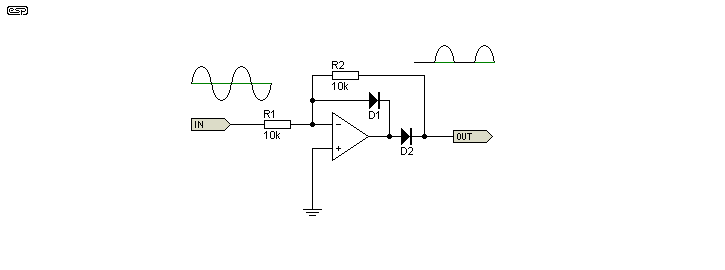Figure 3 - Improved Precision Half Wave Rectifier

This circuit also has its limitations.  The input impedance is now determined by the input resistor, and of course it is more complicated than the basic version.  It must be driven from a low impedance source.  Not quite as apparent, the Figure 3 circuit also has a defined output load resistance (equal to R2), so if this circuit were to be used for charging a capacitor, the cap will also discharge through R2.  Although it would seem that the same problem exists with the simple version as well, R2 (in Figure 1) can actually be omitted, thus preventing capacitor discharge.  Likewise, the input resistor (R1) shown in Figure 1 is also optional, and is needed only if there is no DC path to ground.

Full Wave Precision Rectifiers

Figure 4 shows the standard full wave version of the precision rectifier.  This circuit is very common, and is pretty much the textbook version.  It has been around for a very long time now, and I would include a reference to it if I knew where it originated.  The tolerance of R1, 2, 3, 4 and 5 are critical for good performance, and all five resistors should be 1% or better.  Note that the diodes are connected to obtain a positive rectified signal.  The second stage inverts the signal polarity.  To obtain improved high frequency response, the resistor values should be reduced.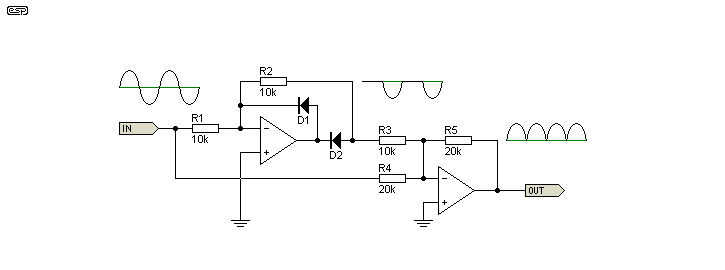Figure 4 - Precision Full Wave Rectifier

This circuit is sensitive to source impedance, so it is important to ensure that it is driven from a low impedance, such as an opamp buffer stage.  Input impedance as shown is 6.66k, and any additional series resistance at the input will cause errors in the output signal.  The input impedance is linear.  As shown, and using TL072 opamps, the circuit of Figure 4 has good linearity down to a couple of mV at low frequencies, but has a limited high frequency response.  Use of high speed diodes, lower resistance values and faster opamps is recommended if you need greater sensitivity and/ or higher frequencies.

The Alternative (Analog Devices)

A little known variation of the full wave rectifier was published by Analog Devices, in Application Brief AB-109 [ 1 ].  In the original, a JFET was used as the rectifier for D2, although this is not necessary if a small amount of low level non-linearity is acceptable.  The resistors marked with an asterisk (*) should be matched, although for normal use 1% tolerance will be acceptable.  C1 may be needed to prevent oscillation.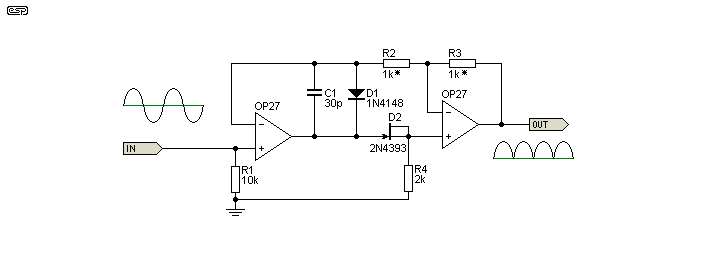Figure 5 - Original Analog Devices Circuit

It was pointed out in the original application note that the forward voltage drop for D2 (the FET) must be less than that for D1, although no reason was given.  As it turns out, this may make a difference for very low level signals, but appears to make little or no difference for sensible levels (above 20mV or so).

Simplified Alternative

For most applications, the circuit shown in Figure 6 will be more than acceptable.  Linearity is very good at 20mV, but speed is still limited by the opamp.  To obtain the best high frequency performance use a very fast opamp and reduce the resistor values.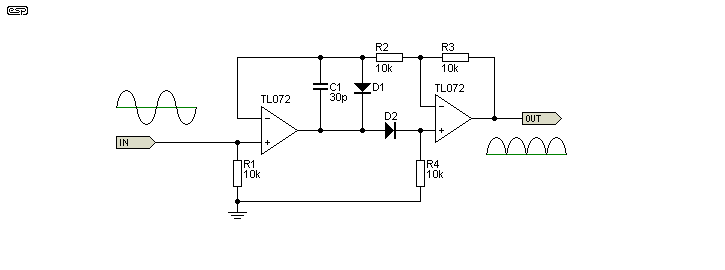Figure 6 - Simplified Version of the AD Circuit

It is virtually impossible to make a full wave precision rectifier any simpler, and the circuit shown will satisfy the majority of low frequency applications.  Where very low levels are to be rectified, it is recommended that the signal be amplified first.  While the use of Schottky (or germanium) diodes will improve low level and/or high frequency performance, it is unreasonable to expect perfect linearity from any rectifier circuit at extremely low levels.  Operation up to 100kHz or more is possible by using fast opamps and diodes.  R1 is optional, and is only needed if the source is AC coupled, so extremely high input impedance (with no non-linearity) is possible.  C1 may be needed to prevent oscillation.

The simplified version shown above (Figure 6) is also found in a Burr-Brown application note [ 3 ].

Another Version

Purely by chance, I found the following variant in a phase meter circuit.  This version is used in older SSL (Solid Stage Logic) mixers, as part of the phase correlation meter.  This circuit exists on the Net in a few forum posts and what appears to be one unauthorised site where several SSL schematics are re-published.  The original drawing I found is dated 1984.  It's also referenced in a Burr-Brown paper from 1973 and an electronics engineering textbook [ 5, 6 ].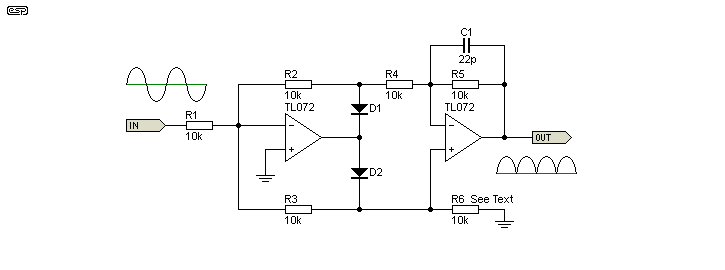Figure 6A - Another Version of the AD Circuit

While it initially looks completely different, that's simply because of the way it's drawn (I copied the drawing layout of the original).  This version is interesting, in that the input is not only inverting, but provides the opportunity for the rectifier to have gain.  The inverting input is of no consequence (it is a full wave rectifier after all), but it does mean that the input impedance is lower than normal ... although you could make all resistor values higher of course.  Input impedance is equal to the value of R1, and is linear as long as the opamp is working well within its limits.

If R1 is made lower than R2 and R3, the circuit has gain.  If R1 is higher than R2 and R3, the circuit can accept higher input voltages because it acts as an attenuator.  For example, if R1 is 1k, the circuit has a gain of 10, and if 100k, the gain is 0.1 (an attenuation of 10).  All normal opamp restrictions apply, so if a high gain is used frequency response will be affected.  C1 is optional - you may need to include it if the circuit oscillates.  The value will normally be between 10pF and 100pF, depending on the speed you need and circuit layout.

One interesting result of using the inverting topology is that the input node is a 'virtual earth' and it enables the circuit to sum multiple inputs.  R1 can be duplicated to give another input, and this can be extended.  The original SSL circuit used two of these rectifiers with four inputs each.  Remember that this is the same as operating the first opamp with a gain of four, so high frequency response may be affected without you realising it.

The circuits shown in Figures 6 and 6A are the simplest high performance full wave rectifiers I've come across, and are the most suitable for general work with audio frequencies.  In most applications, you'll see the Figure 4 circuit, because it's been around for a long time, and most designers know it well.  However, it is definitely not the best performer, and has no advantages over the Figure 6 and 6A simpler alternatives, but it uses more parts and has a comparatively low input impedance.

Another Precision Rectifier (Intersil)

A simple precision rectifier circuit was published by Intersil [ 2 ].  This is an interesting variation, because it uses a single supply opamp but still gives full-wave rectification, with both input and output earth (ground) referenced.  Unfortunately, the specified opamp is not especially common, although other devices could be used.  The CA3140 is a reasonably fast opamp, having a slew rate of 7V/µs.  I will leave it to the reader to determine suitable types (other than that suggested below).  The essential features are that the two inputs must be able to operate at below zero volts (typically -0.5V), and the output must also include close to zero volts.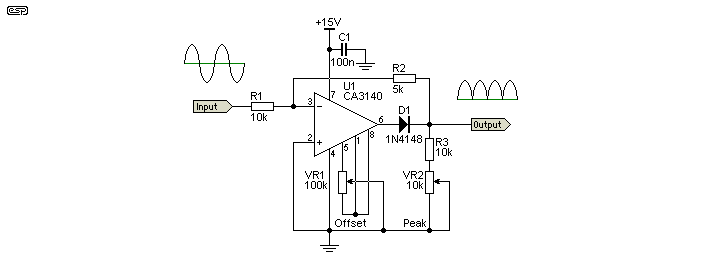Figure 7 - Original Intersil Precision Rectifier Circuit

During the positive cycle of the input, the signal is directly fed through the feedback network to the output.  R3 actually consists of R3 itself, plus the set value of VR2.  The nominal value of the pair is 15k, and VR2 can be usually be dispensed with if precision resistors are used (R3 and VR2 are replaced by a single 15k resistor).

This gives a transfer function of ...

Gain = 1 / ( 1 + (( R1 + R2 ) / R3 )) ... 0.5 with the values shown above

1V input will therefore give an output voltage of 0.5V.  During this positive half-cycle of the input, the diode disconnects the op-amp output, which is at (or near) zero volts.  Note that the application note shows a different gain equation which is incorrect.  The equation shown above works.

During a negative half-cycle of the input signal, the CA3140 functions as a normal inverting amplifier with a gain equal to -( R2 / R1 ) ... 0.5 as shown.  Since the inverting input is a virtual earth point, during a negative input it remains at or very near to zero volts.  When the two gain equations are equal, the full wave output is symmetrical.  Note that the output is not buffered, so the output should be connected only to high impedance stage, with an impedance much higher than R3.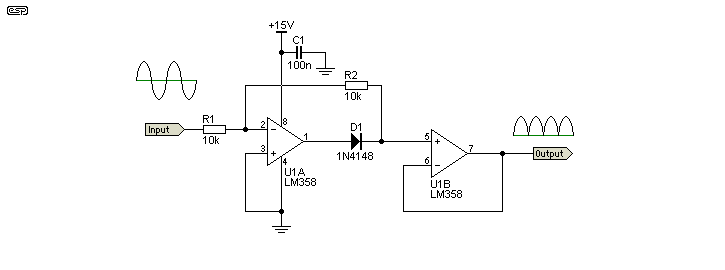Figure 8 - Modified Intersil Circuit Using Common Opamp

Where a simple, low output impedance precision rectifier is needed for low frequency signals (up to perhaps 10kHz as an upper limit), the simplified version above will do the job nicely.  It does require an input voltage of at least 100mV, because there is no DC offset compensation.  Because the LM358 is a dual opamp, the second half can be used as a buffer, providing a low output impedance.  Minimum suggested input voltage is around 1V peak (710mV), which will give an average output voltage of 320mV.  Higher input voltages will provide greater accuracy, but the maximum is a little under 10V RMS with a 15V DC supply as shown.  The LM358 is not especially fast, but is readily available at low cost.

Limitations:   Note that the input impedance of this rectifier topology is non-linear.  The impedance presented to the driving circuit is 30k for positive half cycles, but only 10k for negative half-cycles.  This means that it must be driven from a low impedance source - typically another opamp.  This increases the overall complexity of the final circuit.

Single Supply Precision Rectifier (B-B/ TI)

An interesting variation was shown in a Burr-Brown application note [ 3 ].  This rectifier operates from a single supply, but accepts a normal earth (ground) referenced AC input.  The only restriction is that the incoming peak AC signal must be below the supply voltage (typically +5V for the OPA2337 or OPA2340).  The opamps used must be rail-to-rail, and the inputs must also accept a zero volt signal without causing the opamp to lose control.

The circuit is interesting for a number of reasons, not the least being that it uses a completely different approach from most of the others shown.  The rectifier is not in the main feedback loop like all the others shown, but uses an ideal diode (created by U1B and D1) at the non-inverting input, and this is outside the feedback loop.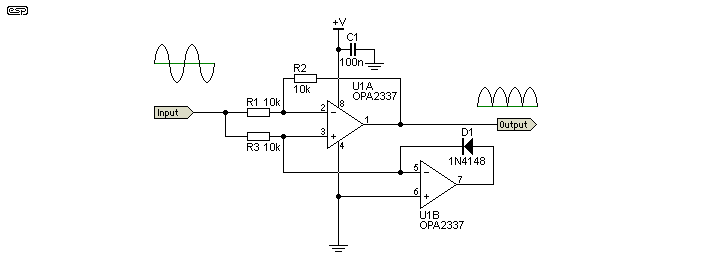Figure 9 - Burr-Brown Circuit Using Suggested Opamp

For a positive-going input signal, the opamp (U1A) can only function as a unity gain buffer, since both inputs are driven positive.  Both the non-inverting and inverting inputs have an identical signal, a condition that can only be achieved if the output is also identical.  If the output signal attempted to differ, that would cause an offset at the inverting input which the opamp will correct.  It is worth remembering my opamp rules described at the beginning of this app. note.

For a negative-going input signal, The ideal diode (D1 and U2B) prevents the non-inverting input from being pulled below zero volts.  Should this happen, the opamp can no longer function normally, because input voltages are outside normal operating conditions.  The opamp (U1A) now functions as a unity gain inverting buffer, with the inverting input maintained at zero volts by the feedback loop.  If -10µA flows in R1, the opamp will ensure that +10uA flows through R2, thereby maintaining the inverting input at 0V as required.

Limitations:   Input impedance is non-linear, having an almost infinite impedance for positive half-cycles, and a 5k input impedance for negative half-cycles.  The input must be driven from an earth (ground) referenced low impedance source.  Capacitor coupled sources are especially problematical, because of the widely differing impedances for positive and negative going signals.  The maximum source resistance for a capacitor-coupled signal input is 100 ohms for the circuit as shown (one hundredth of the resistor values used for the circuit), and preferably less.  The capacitance is selected for the lowest frequency of interest.

Simple Full Wave Rectifier

This rectifier is something of an oddity, in that it is not really a precision rectifier, but it is full wave.  It is an interesting circuit - sufficiently so that it warranted inclusion even if no-one ever uses it.  This rectifier was used as part of an oscillator [ 4 ] and is interesting because of its apparent simplicity and wide bandwidth even with rather pedestrian opamps.

A simulation using TL072 opamps indicates that even with a tiny 5mV peak input signal (3.5mV RMS) the frequency response extends well past 10kHz but for low level signals serious amplitude non-linearity can be seen.  The original article didn't even mention the rectifier, and no details were given at all.  However, I have been able to determine the strengths and weaknesses by simulation.  Additional weaknesses may show up in use of course.  A reader has since pointed out something I should have seen (but obviously did not) - R3 should not be installed.  Without R3, linearity is far better than expected.

It's not known why R3 was included in the original JLH design, but in the case of an oscillator stabilisation circuit it's a moot point.  The circuit will always have more or less the same input voltage, and voltage non-linearity isn't a problem.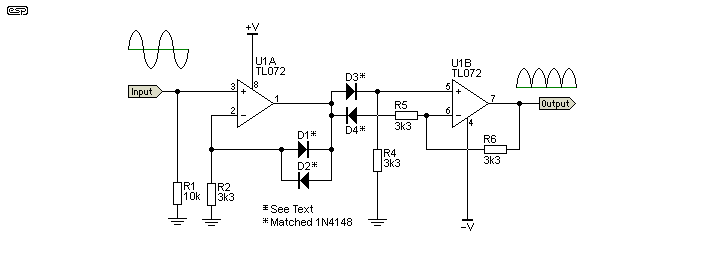Figure 10 - Simple Precision Full Wave Rectifier

One thing that is absolutely critical to the sensible operation of the circuit at low signal levels is that all diodes must be matched, and in excellent thermal contact with each other.  The actual forward voltage of the diodes doesn't matter, but all must be identical.  The lower signal level limit is determined by how well you match the diodes and how well they track each other with temperature changes.

The first stage allows the rectifier to have a high input impedance (R1 is 10k as an example only).  Nominal gain as shown is 1 (with R3 shorted).  R3 was included in the original circuit, but is actually a really bad idea, as it ruins the circuit's linearity.  Without it, the circuit is very linear over a 60dB range.  This is more than enough for any analogue measurement system.

Limitations:   Linearity is very good, but the circuit requires closely matched diodes for low level use because the diode voltage drops in the first stage (D1 & D2) are used to offset the voltage drops of D3 & D4.  At input voltages of more than a volt or so, the non-linearities are unlikely to cause a problem, but diode matching is still essential (IMO).  Low level performance will be woeful if accurate diode forward voltage and temperature matching aren't up to scratch.  A forward voltage difference of only 10mV between any two diodes will create an unacceptable error.  The overall linearity is considerably worse if R3 is included.

Simple capacitor smoothing cannot be used at the output because the output is direct from an opamp, so a separate integrator is needed to get a smooth DC output.  This applies to most of the other circuits shown here as well and isn't a serious limitation.

Simple Full Wave Meter Amplifier

The final circuit is a precision full-wave rectifier, but unlike the others shown it is specifically designed to drive a moving coil meter movement.  There is no output voltage as such, but the circuit rectifies the incoming signal and converts it to a current to drive the meter.  This general arrangement is (or was) extremely common, and could be found in audio millivoltmeters, distortion analysers, VU meters, and anywhere else where an AC voltage needed to be displayed on a moving coil meter.  Digital meters have replaced it in most cases, but it's still useful, and there are some places where a moving coil meter is the best display for the purpose.  This type of rectifier circuit is discussed in greater detail in AN002.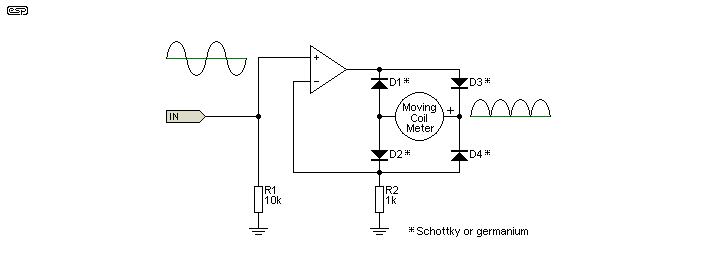Figure 11 - Moving Coil Meter Amplifier

The circuit is a voltage to current converter, and with R2 as 1k as shown, the current is 1mA/V.  If a 1V RMS sinewave is applied to the input, the meter will read the average, which is 900µA.  Adjusting R2 varies the sensitivity, and changing R2 to 900 ohms means the meter will show 1mA for each volt (RMS) at the input.  This assumes a meter with a reasonably low resistance coil, although in theory the circuit will compensate for any series resistance.

This type of circuit almost always has R2 made up from a fixed value and a trimpot, so the meter can be calibrated.  Although shown with an opamp IC, the amplifying circuit will often be discrete so that it can drive as much current as needed, as well as having a wide enough bandwidth for the purpose.  Millivoltmeters and distortion analysers in particular often need an extended response (100kHz or more is common), and few opamp ICs are able to provide a wide enough bandwidth to work well with anything much over 15kHz.  The problem is worse at low levels because the opamp output has to swing very quickly to overcome the diode forward voltage drop.  It's common to use a capacitor in parallel with the movement to provide damping, but that also changes the calibration.

Limitations:   The output is very high impedance, so the meter movement is not damped unless a capacitor is used in parallel.  The meter will then show the peak value which might not be desirable, depending on the application.

As already noted, the opamp needs to be very fast.  Linearity is good provided the amplifier used has high bandwidth.  The circuit works better with low-threshold diodes (Schottky or germanium for example), which do not need to be matched because the circuit relies on current, and not voltage.  It also only works as intended with a moving coil meter and is not suited to driving digital panel meters or other electronic circuits.  It can be done, but there's no point as the circuit would be far more complex than others shown here.

Conclusion

Although the waveforms and tests described above were simulated, the Figure 6 circuit was built on my opamp test board.  This board uses LM1458s - very slow and extremely ordinary opamps, but the circuit operated with very good linearity from below 20mV up to 2V RMS, and at all levels worked flawlessly up to 35kHz using 1k resistors throughout.  Variations of Figure 11 have been used in several published projects and in test equipment I've built over the years

One thing that became very apparent is that the Figure 6 circuit is very intolerant of stray capacitance, including capacitive loading at the output.  Construction is therefore fairly critical, although adding a small cap (as shown in Figures 5 & 6) will help to some extent.  I don't know why this circuit has not overtaken the 'standard' version in Figure 4, but that standard implementation still seems to be the default, despite its many limitations.  Chief among these are the number of parts and the requirement for a low impedance source, which typically means another opamp.  The impedance limitation does not exist in the alternative version, and it is far simpler.

The Intersil and Burr-Brown alternatives are useful, but both have low (and non-linear) input impedance.  They do have the advantage of using a single supply, making both more suitable for battery operated equipment or along with logic circuitry.  Remember that all versions (Figures 7, 8 & 9) must be driven from a low impedance source, and the Figure 7 circuit must also be followed by a buffer because it has a high output impedance.

In all, the Figure 6 circuit is the most useful.  It is simple, has a very high (and linear) input impedance, low output impedance, and good linearity within the frequency limits of the opamps.  The Figure 6A version is also useful, but has a lower input impedance and requires 2 additional resistors (R1 in Figure 6 is not needed if the signal is earth referenced).

The above circuits show just how many different circuits can be applied to perform (essentially) the same task.  Each has advantages and limitations, and it is the responsibility of the designer to choose the topology that best suits the application.  Not shown here, but just as real and important, is a software version.  Digital signal processors (DSPs) are capable of rectification, conversion to RMS and almost anything else you may want to achieve, but are only applicable in a predominantly digital system.

With all of these circuits, it's unrealistic to expect more than 50dB of dynamic range with good linearity.  This gives a range from 10mV up to 3.2V (peak or RMS) with supplies of ±12-15V.  Use of precision high speed opamps may increase that, but if displayed on an analogue (moving coil) meter, you can't read that much range anyway - even reading 40dB is difficult.  100:1 (full scale to minimum) is not easily read on most analogue movements - even assuming that the movement itself is linear at 100th of its nominal FSD current.

Many of the circuits shown have low impedance outputs, so the output waveform can be averaged using a resistor and capacitor filter.  The value appearing across the filter cap is the average of the rectified signal - for a sinewave, the average is calculated by ...

VAVG = ( 2 * VPeak ) / π       or ...
VAVG = VPeak * 0.637

It turns out that the RMS value of a sinewave is (close enough to) the average value times 1.11 (the inverse is 0.9) and this makes it easy enough to convert one to another.  However, it only gives an accurate reading with a sinewave, and will show serious errors with more complex waveforms.  To see just how much error is involved, see AN012 which covers true RMS conversion techniques and includes a table showing the error with non-sinusoidal waveforms.

References
1. Analog Devices, Application Briefs, AB-109, James Wong.
2. Intersil CA3140/CA3140A Data Sheet (Datasheet Application Note, 11 July 2005, Page 18), Intersil CA3140
3. SBOA068 - Precision Absolute Value Circuits - By David Jones and Mark Stitt, Burr-Brown (now Texas Instruments)
4. Wien-Bridge Oscillator With Low Harmonic Distortion, J.L. Linsley-Hood, Wireless World, May 1981
5. Applications of Operational Amplifiers, Third Generation Techniques - Jerald Graeme, Burr-Brown, 1973, pp. 123-124
6. Microelectronics: Digital and Analog Circuits and Systems (International Student Edition), Author: Jacob Millman, Publisher: McGraw Hill, 1979 (Chapter 16.8, Fig. 16-27)App. Notes IndexMain Index

 Copyright Notice.This article, including but not limited to all text and diagrams, is the intellectual property of Rod Elliott, and is Copyright © 2004 - 2009.  Reproduction or re-publication by any means whatsoever, whether electronic, mechanical or electro-mechanical, is strictly prohibited under International Copyright laws.  The author (Rod Elliott) grants the reader the right to use this information for personal use only, and further allows that one (1) copy may be made for reference while constructing the project.  Commercial use is prohibited without express written authorisation from Rod Elliott.  Referenced material is Copyright - see original material for details.
Page Created and Copyright © Rod Elliott 02 Jun 2005./ Updated 23 July 2009 - added Intersil version and alternative./ 27 Feb 2010 - included opamp rules and BB version./ Jan 2011 - added figure 10, text and reference./ Mar 2011 - added Fig 6A and text./ Aug 2017 - extra info on Figure 10 circuit, and added peak-average formula.